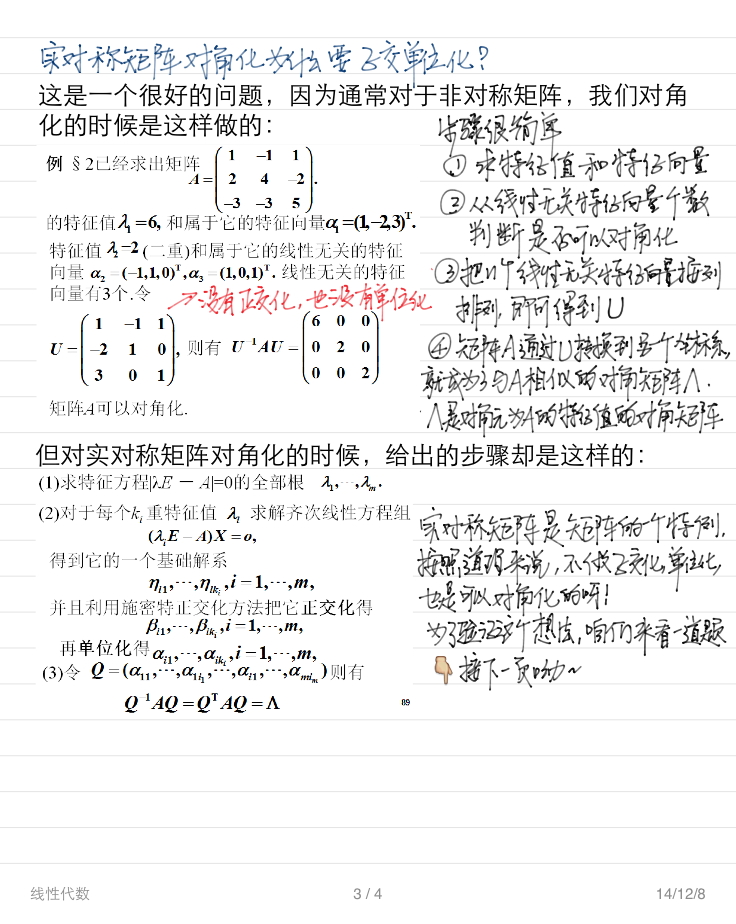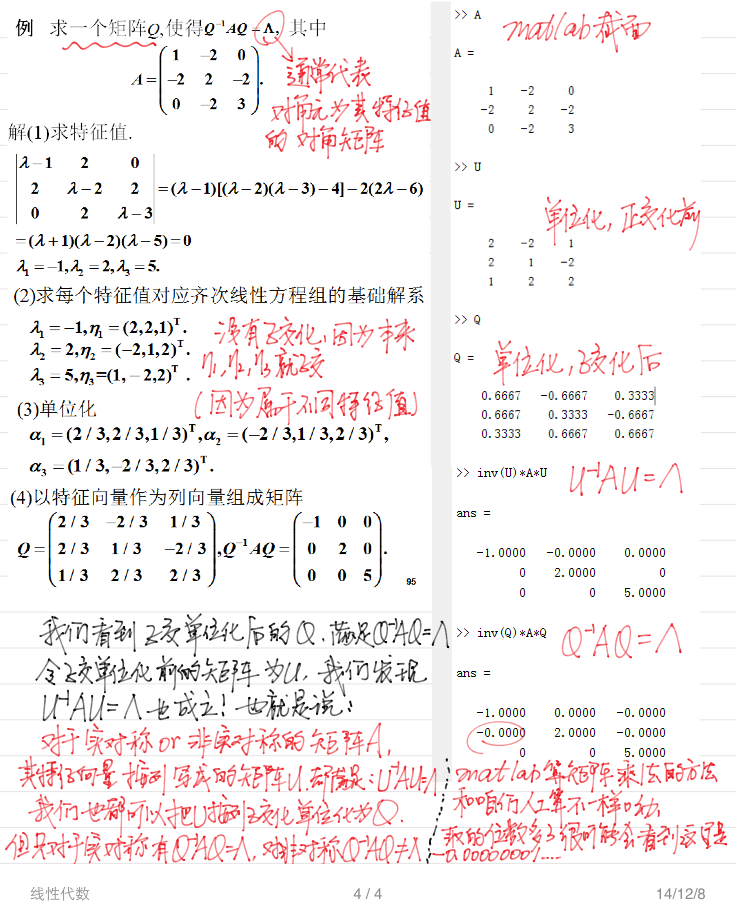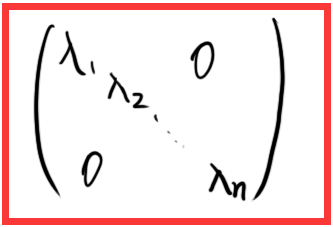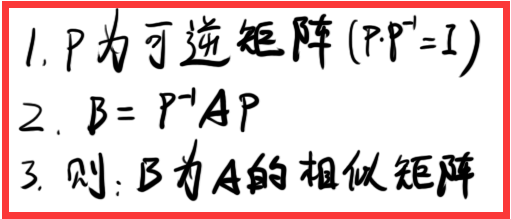• 今天晚上王小民同学问了助教姐姐一个问题，为什么对一个一般的矩阵对角化的时候，我们不用做正交单位化，对实对称矩阵对角化的时候却要做呢？这是一个很好的问题，所以和大家分享一下。 最后的结论就是...
今天晚上王小民同学问了助教姐姐一个问题，为什么对一个一般的矩阵对角化的时候，我们不用做正交单位化，对实对称矩阵对角化的时候却要做呢？这是一个很好的问题，所以和大家分享一下。最后的结论就是：如果不做正交单位话，我们一样可以通过U（把特征向量按照列写成的矩阵），把一个实对称矩阵对角化为以它的特征值为对角元的对角矩阵。

我们知道，对应一个特征值的特征向量乘以任何一个非零的系数，仍然还是对应着这个特征值的特征向量，如果一个特征值对应多个特征向量，那在它们张成的空间里找出同样数量的线性不相关的向量，也都是这个特征值的特征向量，所以说特征向量并不唯一，也就是说这里的U是不唯一的。

而对于一个实对称矩阵，它的属于不同特征值的特征向量天生就是正交的，这使得我们只要在每个特征值内部选取合适的互相正交的特征向量，就能保证所有的特征向量都正交。而我们刚刚说过，特征向量乘以一个系数，仍然还是特征向量。所以，对于实对称矩阵来说，我们完全可以在诸多的U中选出一个特殊的Q，让Q的每一个列向量都互相正交而且长度为1。这时我们就惊喜的发现，这样的相当于由一组标准正交基当做列向量组成的矩阵Q，正是一个正交矩阵。

于是，我们就清楚的知道了，对实对称矩阵对角化的时候，正交单位化不是必须的，只有当我们想在实对称矩阵的诸多U里选取一个正交矩阵Q时，才需要做。正交矩阵有很多很好的性质，于是乎想从U里找到一个Q也变得情有可原了不是？

展开全文• 对称矩阵对角化，有两篇论文，内含Matlab实现代码，在文章里的，可以直接写下来使用。测试过，还可以。
• 假设两个实对称矩阵A和B,如果存在一个可逆的矩阵X， XAX'=B,已知A和B,知道怎么用matlab求X? 本例中数据如下： A=[0.287402 0 0  0 0.483209 0  0 0 0.000025]; B=[0.287402 -0.028039 -0.0000727  -0....
假设两个实对称矩阵A和B,如果存在一个可逆的矩阵X，XAX'=B,已知A和B,知道怎么用matlab求X?
本例中数据如下：
A=[0.287402 0 0
0 0.483209 0
0 0 0.000025];
B=[0.287402 -0.028039 -0.0000727
-0.028039 0.483209 0.001299
-0.0000727 0.001299 0.000025];
下面是对一个对称矩阵求转换后的对角矩阵的matlab程序
程序来自
《基于Matlab的实对称矩阵对角化》一文，作者 计文军等
其中a的对称矩阵，d是对角化后的矩阵，p是相应的合同变换，满足pTap=d
function [p,d]=juzheng(a)
[m,n]=size(a);
a=[a eye(n)]';
for k=1:n
if a(k,k)==0
for r=(k+1):n
if a(k,r)~=0
for i=k:n
a(k,i)=a(k,i)+a(r,i);
end
for i=k:2*n
a(i,k)=a(i,k)+a(i,r);
end
break
end
end
end

for i=k+1:n
l=a(i,k)/a(k,k);
for j=k:n
a(i,j)=a(i,j)-l*a(k,j);
end
for j=k:2*n
a(j,i)=a(j,i)-l*a(j,k);
end
end

end
p=a(n+1:2*n,1:n);
d=a(1:n,1:n);
return

下面在脚本文件中调用juzheng.m函数
A=[0.287402 0 0
0 0.483209 0
0 0 0.000025];
B=[0.287402 -0.028039 -0.0000727
-0.028039 0.483209 0.001299
-0.0000727 0.001299 0.000025];
[p,d]=juzheng(B);
X=(inv(p))';% 这一步是将计算的结果转成本例中我所需要的形式%你可以验证X*A*X'=B

完毕！
感谢文章作者

展开全文• 原来，实对称矩阵对角化是为解决解析几何中二次曲面是何类型而提出的，因为二次曲面的方程可以写成(x,y,z)A(xyz)(x,y,z)A\begin{pmatrix}x\\y\\z\end{pmatrix}(x,y,z)A⎝⎛​xyz​⎠⎞​的形式，而这里的A就是一个实...
文章目录正交相似定理1证明定理2证明定理3证明命题1命题2
原来，实对称矩阵对角化是为解决解析几何中二次曲面是何类型而提出的，因为二次曲面的方程可以写成$(x,y,z)A\begin{pmatrix}x\\y\\z\end{pmatrix}$的形式，而这里的A就是一个实对称矩阵，为了更好的看出曲线的类型，需要把二次项给消掉，使得变成$(x^*,y^*,z^*)T^{-1}AT\begin{pmatrix}x^*\\y^*\\z^*\end{pmatrix}$的形式，而$T^{-1}AT$是一个对角矩阵。不过为什么这里的T一定是正交矩阵？虽然后面证明了能将实对称矩阵对角化的可逆矩阵一定是正交矩阵，不过这里好像还有一个从几何上证明的定理？？
实对称矩阵是指：实数域上的对称矩阵
正交相似

大概就是对于矩阵$A,B$，若存在一正交矩阵$T$使得$T^{-1}AT=B$就说$A$正交相似于$B$
正交相似是$n$级实矩阵组成的集合的一个等价关系，拥有以下三个性质

反身性
对称性
传递性

定理1
实对称矩阵的特征多项式的每一个复根都是实数，从而它们都是特征值。
证明

设$\lambda_0$是$n$级实对称矩阵A的任一复根,只需证明$\bar{\lambda_0}=\lambda_0$，便能证明其为实数
把A看成复矩阵，从而$\exist\alpha\in C^n，且\alpha\ne 0$，有$A\alpha=\lambda_0\alpha\tag{1}$
由于A是实矩阵，所以$\bar{\lambda_0}$也是A的一个特征值，$\bar{\alpha}$是$\bar{\lambda_0}$的一个特征向量（$P_{273}例2$：$A$是复数域上的n级实矩阵,若虚数$\lambda_0$是A的属于$\lambda_0$的一个特征向量，那么$\bar{\lambda_0}$也是A的一个特征值，且特征向量为$\bar{\alpha}$）
所以就有$A\bar{\alpha}=\bar{\lambda_0}\bar{\alpha}$对其转置有$\bar{\alpha}'A'=\bar{\lambda_0}\bar{\alpha}'$在上式右乘$\alpha$,得$\bar{\alpha}'A'\alpha=\bar{\lambda_0}\bar{\alpha}'\alpha\tag{2}$
在(1)式左边乘上$\bar{\alpha}'$，得$\bar{\alpha}'A\alpha=\lambda_0\bar{\alpha}'\alpha\tag{3}$
(2)=(3)$\Rightarrow$ $(\bar{\lambda_0}-\lambda_0)\bar{\alpha}'\alpha=0$ $\bar{\alpha}'\alpha\ne0$,So,$\bar{\lambda_0}=\lambda_0$

定理2
实对称矩阵的不同特征值的特征向量是正交的
证明

设$\lambda_1,\lambda_2$分别是A的两个不同的特征值，$\alpha,\beta$分别是它们 的一个特征向量$A\alpha=\lambda_1\alpha\tag{1}$ $A\beta=\lambda_2\beta\tag{2}$要证$\alpha'\beta=0$
让（1）转置，得$\alpha'A=\lambda_1\alpha'$右乘$\beta$，得$\alpha'A\beta=\lambda_2\alpha'\beta=\lambda_1\alpha'\beta$ $\Rightarrow$ $\alpha'\beta=0$
是不是觉得哪里有点奇怪，$\alpha'A\beta=\lambda_2\alpha'\beta$这样可以吗，不是矩阵相乘才有结合律吗?
啧啧啧，一看就是矩阵运算没有学好，刚刚翻到$P_{143}$矩阵的运算，第一个性质就是说的矩阵的结合律！
设$A=(a_{ij})_{s×n},B=(b_{ij})_{n×m},C=(c_{ij})_{m×r}$，则满足$(AB)C=A(BC)$

定理3
实对称矩阵一定正交相似于对角矩阵
这表明$\Rightarrow$实对称矩阵一定可对角化（真是上帝的宠儿，一出生就自带可对角化的光环）
证明
这个证明有难度了，从一开始就意想不到

首先，这里用的是数学归纳法
$n=1$时，$(1)^{-1}(a)(1)=(a)$，命题真
假设对$n-1$级矩阵都满足以上命题，现在看$n$级实对称矩阵A

由定理1得，实对称矩阵必有特征值，故取A的一个特征值$\lambda_1$,再取$\lambda_1$的一个特征向量$\eta_1$，取的$\eta_1$要满足$|\eta_1|=1$
然后！！把$\eta_1$扩充为$R^n$的一个基，经过施密特正交化和标准化，得到$R^n$的一组标准正交鸡$\eta_1,\eta_2,...,\eta_n$,令$T_1=(\eta_1,\eta_2,...,\eta_n)$则$T_1$是正交矩阵
于是$T_1^{-1}AT_1=T_1^{-1}(A\eta_1,A\eta_2,...,A\eta_n)$ $=(\lambda_1T_1^{-1}\eta_1,T_1^{-1}A\eta_2,...,T_1^{-1}A\eta_n)$由于正交矩阵有$T'=T^{-1}$，所以$T_1^{-1}=T_1'=\begin{pmatrix}\eta_1'\\\eta_2'\\...\\\eta_n'\end{pmatrix}$ 所以$T_1^{-1}\eta_1=\begin{pmatrix}1\\0\\...\\0\end{pmatrix}=\varepsilon_1$
所以$T_1^{-1}AT_1=\begin{pmatrix}\lambda_1&\alpha\\0&B\end{pmatrix}$由于A是实对称，则$T_1^{-1}AT_1$也是实对称（正交矩阵节$P_{218}$第一题就证明的是这个！！）$\Rightarrow \alpha=0$，所以$T_1^{-1}AT_1=\begin{pmatrix}\lambda_1&0\\0&B\end{pmatrix}$B也是实对称矩阵

由设，得$\exist 正交矩阵T_2$，使得$T_2^{-1}BT_2=diag\{\lambda_2,...,\lambda_n\}$
所以，只需令$T=T_1\begin{pmatrix}1&0\\0&T_2\end{pmatrix}$就有$T^{-1}AT=diag\{\lambda_1,\lambda_2,...,\lambda_n\}$

命题1

若矩阵A是$n$阶实矩阵
且A正交相似于一个对角矩阵D
$\Rightarrow$A绝对是实对称矩阵

所以只有实对称矩阵才有正交相似的权利，非常霸道了
（有一个蒙面人说，我是实的且能正交相似，你们知道我是谁吗？）
（众人：哎哟你可拉到吧！还蒙面呢，只有实对称矩阵才能这样，你肯定是实对称矩阵家族的！）
命题2
$A,B$是两个实对称矩阵,则
$A,B相似\Leftrightarrow A,B正交相似$


展开全文• 1 ，对称矩阵 ： 定于 ： 1 ，如果 ：矩阵 A = A 的转置 2 ，那么 ：A 为对称矩阵 如图 ： 2 ，对角矩阵 ： 定义 ： 1 ，主对角线的元素不为 0 2 ，其他元素都为 0 ...5 ，对角化 ： ...2 ，则称这个过程是对角化
1 ，对称矩阵 ：

定于 ：
1 ，如果 ：矩阵 A = A 的转置
2 ，那么 ：A 为对称矩阵
如图 ：2 ，对角矩阵 ：

定义 ：
1 ，主对角线的元素不为 0
2 ，其他元素都为 0
例如 ：3 ，正定矩阵 ：

定义 ：
1 ，可以让非零实向量乘以他自己的转置 > 0 ，这样的矩阵叫正定矩阵
2 ，理解 ： 把它的方向正过来
如图 ：4 ，相似矩阵 ：

定义 ：5 ，对角化 ：

定义 ：
1 ，如果一个矩阵的像是矩阵是一个对角矩阵
2 ，则称这个过程是对角化


展开全文线性代数
• 而考试中考察的三阶实对称矩阵对角化基本都是三阶的。而且正常情况下特征根一定是整数。因此基于此，有一些特殊的方法可以快速计算三阶实对称矩阵的特征值和特征向量。 一. 猜根法计算特征值 特征值之和等于...
• 对称矩阵相似对角化Matlab程序，用到的朋友可以下载看看。
• Note：PCA主成分分析用到实对称阵的相似对角化。1.对角阵概念2.矩阵与对角阵相似的条件3.一般矩阵的相似对角化4.实对称矩阵的相似对角化5.协方差矩阵的相似对角化(end)...
• 每个元素都为实数的对角矩阵称为实对称矩阵，实对称矩阵必定相似于一个对角矩阵（对角线以外的元素全为0的矩阵），即存在可逆矩阵P，使得，且存在正交矩阵Q，使得 实对称矩阵化为对角矩阵的步骤： 1.找出全部特征...
• ## 对称矩阵的对角化

千次阅读 2019-03-29 09:32:22
• 在极分解的证明中使用过此定理，证明于此。 埃尔米特矩阵是指复对称矩阵，实对称矩阵是其特例。 转载于:https://www.cnblogs.com/zhixingr/p/8750210.html...
• 文章目录实对称矩阵对称变换实对称矩阵的正交对角化参考 本节目的: 讨论一类必可相似对角化的矩阵: 实对称矩阵. 证明: 若 AAA 是 nnn 阶实对称矩阵, 则 (1) AAA 的特征值都是实数. (2) 互异特征值的特征向量必然...
• 对称矩阵一定可以对角化. 最近看共轭梯度下降的时候看到有人的推导里面用到了这个命题. 虽然以前学过, 但是学得很渣, 所以没有自己想过这个命题怎么样成立的. 现在将这些证明过程梳理一下. 实对称矩阵含有n个实根 ...
• 4.一定可以用正交矩阵相似对角化（满足的矩阵为正交阵），步骤如下 （1）求A的特征值λ1、λ2、λ3 （2）求特征向量α1、α2、α3 （3）改造特征向量 a. 如λi≠λj 只需要单位化 ...
• 对于实对称矩阵AAA，有J=P−1APJ=P^{-1}APJ=P−1AP,JJJ为AAA的若当标准型。 而 JT=PTAP−T=PTPP−1APP−1(P−1)T=(PTP)J(PTP)−1J^{T}=P^{T}AP^{-T}=P^TPP^{-1}APP^{-1}(P^{-1})^T=(P^TP)J(P^TP)^{-1}JT=PTAP−T=...
• 为什么实对称矩阵的相似对角化要用正交矩阵？ 一般矩阵的相似对角化用它的特征向量组成的矩阵就可以了，为什么实对称矩阵的相似对角化这么特殊呢，名称叫做正交矩阵化，求得特征向量矩阵后还要正交化和单位化使之...
• Ae=λe Ae=\lambda e\newline Ae=λe 取所有特征值 和特征向量，用Λ\LambdaΛ表示对角线为特征值，其余位置...可完成 对称 矩阵对角化，如下： A=EΛE−1=EΛET A=E\Lambda E^{-1}=E\Lambda E^{T} A=EΛE−1=E...
• n阶矩阵A可正交对角化的充分条件是A是实对称矩阵，即若A是实对称矩阵则A必可正交对角化。 首先，有以下定理： 若的特征值为，且，则存在正交矩阵Q，使A相似于如下三角矩阵： 证明如下（数学归纳法）： 设n*n阶...正交相似
• 因此，此提交有助于人们使用 Hermitian/对称矩阵的三对角化，A = Q * T * Q'， 其中 A 是 Hermitian 或对称的，T 是实对称三对角线，Q 是酉或正交的。 最初的 Lapack 接口例程来自 Tim Toolan 在“文件交换”中的...matlab
• 对称不定矩阵实现三对角分解 PAPT = LTLT的关键问题是如何从 Tk- 1约化到 ...以矩阵表示的待定元素为直接运算结果,确定 Tk- 1矩阵表示的递进过程,逐步约化得最终的矩阵对角化结果 T,从而代替矩阵本身繁琐的直接运算.
• 不一定，可以直接用一般矩阵的方法求其对角阵，即可以不用正交单位化，直接用【p逆Ap=A的对角阵】来做，书上一上来就说用正交阵来对角化就是淡村为了体现这个方法而已，但是还是有好处的，比如正交单位化后，要求p逆...
• matlab开发-热对称矩阵在LaPackInterface上的三对角化。代码通过调用lapack例程来计算Hermitian矩阵的三对角分解。
• 以主对角线为对称矩阵MATLAB代码联合对角化的拟牛顿算法 文件和网站 有关文档和示例，请参见此处： 概括 该Python软件包包含用于对一组正定对称矩阵进行快速对角对角化的代码。 主要功能是qndiag ，它以一组大小为(p...
• 1. 对任一实对称矩阵，存在正交矩阵，满足矩阵的连乘等于对角矩阵 2. 求正交矩阵与对角矩阵的计算步骤 3. 实对称矩阵的正交矩阵与对角矩阵的求解示例 4. 两个实对称矩阵相似的充要条件是它们有...
• 矩阵对角化：A=SΛS−1A=S \Lambda S^{-1}A=SΛS−1 AT=(S−1)TΛSTA^{\mathrm{T}}=\left(S^{-1}\right)^{\mathrm{T}} \Lambda S^{\mathrm{T}}AT=(S−1)TΛST 如果 A=ATA=A^{\mathrm{T}}A=AT， SΛS−1=(S−1)TΛSTS...线性代数
• 【第一章 线性代数】1.7矩阵对角化二次型 在线LaTeX公式编辑器 任务详解： 1、掌握相似矩阵，对角化，对角化的条件。对称矩阵一定可以对角化 2、二次型与矩阵的正定性，以及如何判断正定，可逆的又一种判断方法...
• 文章目录相似矩阵矩阵的对角化对称矩阵对角化对称阵A对角化的步骤 相似矩阵 设A，B都是n阶矩阵，若有可逆矩阵P，使 P−1AP=B,P^{-1}AP=B,P−1AP=B,则称B是A的相似矩阵，或说矩阵A与B相似，对A进行运算P−1APP^{-1...线性代数...